# Bernstein algebra

(diff) ← Older revision | Latest revision (diff) | Newer revision → (diff)
Around 1900, S.N. Bernstein (cf. [a3], [a4], [a5]) worked on an important problem concerning the laws of formal genetics. This problem is known today as the Bernstein problem. Following Yu.I. Lyubich (cf. [a10]), this problem can be expressed as follows. The state of a population in a given generation is described by a vector inwhose coordinates satisfy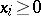() and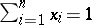. The setof all states is a simplex in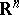and the vertices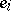() ofare the different types of individuals in the population. If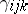is the probability that an individualappears in the next generation from parents of typesand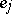, then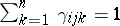(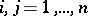) and(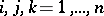). In absence of selection and under random hypothesis, the state of the population in the next generation can be written, in terms of coordinates, as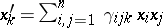(). These relations define a quadratic operator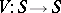called the evolutionary quadratic operator. The Bernstein stationarity principle says that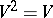and the Bernstein problem aims at describing all quadratic operators satisfying this principle. Bernstein solved his problem for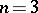and much progress was achieved recently (cf. [a6], [a8]) in this direction. The Bernstein problem can be translated in terms of algebra structure. In fact, overan algebra structure can be defined via the operatorby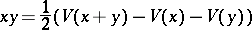for all, and if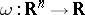is the mapping defined by, thenif and only if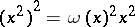for all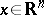. Moreover,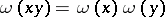for all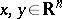. Of course, to define this multiplication over the whole spacestarting from the simplex, one has to make convenient extensions of this multiplication by bilinearity. Now, in general, ifis a (commutative) field andis a commutative-algebra, then a weighted algebra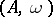overis said to be a Bernstein algebra iffor all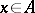(cf. [a2]). In recent years (1990s), the theory of Bernstein algebras has been substantially improved. V.M. Abraham (cf. [a1]) suggests the construction of a generalized Bernstein algebra. In this perspective, for an element, whereis a weighted algebra, the plenary powersofare defined by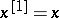andfor all integer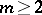. The plenary powers can be interpreted by saying that they represent random mating between discrete non-overlapping generations.is called anth order Bernstein algebra if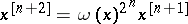for all, where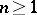is the smallest such integer (cf. [a11]). Second-order Bernstein algebras are simply called Bernstein algebras and first-order Bernstein algebras are also called gametic diploid algebras. The interpretation of the equation(such that) is that equilibrium in the population is reached after exactlygenerations of intermixing. For genetic properties of Bernstein algebras, see [a7] and [a12].